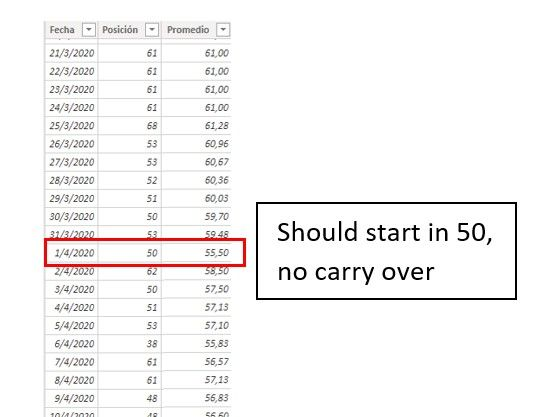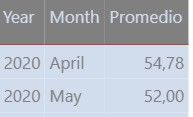cancel
Showing results for
Did you mean:Anonymous
Not applicable

## Average per row, reseting on each month

Hello!

First Post.

I have data of my country's position on a ranking list.

I need to determine the average of that position for each month separately.

Currently im using:

Promedio = CALCULATE(
AVERAGE(PowerBI[Posición]);
FILTER(PowerBI;
PowerBI[Fecha].[Month]<=EARLIER(PowerBI[Fecha].[Month])
&& PowerBI[Fecha].[Day]<=EARLIER(PowerBI[Fecha].[Day]))
)

But the averages are wrongly calculated.
I also noticed that on the first day of the month if position is 50 the average shows 55.5 which means its carrying values from the previous months.1 ACCEPTED SOLUTIONCommunity Support

Hi @Anonymous ,

We can use the following steps to meet your requirement.

1. Create two calculate columns, one is year, another is month.

``````Month = MONTH('Table'[Date])

year = YEAR('Table'[Date])``````2. Then we can create a measure,

``````Each month AVG =
var current_month = MAX('Table'[Month])
var Total_value = CALCULATE(SUM('Table'[value]))
var Month_day = CALCULATE(DISTINCTCOUNT('Table'[Date]),FILTER('Table','Table'[Month] = current_month))
return
DIVIDE(Total_value,Month_day)``````

The result like this,3. Or we can create a calculate column,

``````AVG column =
var total_month = CALCULATE(DISTINCTCOUNT('Table'[Date]),FILTER(ALLSELECTED('Table'),'Table'[Month]=EARLIER('Table'[Month])&& 'Table'[year]=EARLIER('Table'[year])))
var current_month = CALCULATE(SUM('Table'[value]),FILTER(ALLSELECTED('Table'),'Table'[Month]=EARLIER('Table'[Month]) && 'Table'[year]=EARLIER('Table'[year])))
return
DIVIDE(current_month,total_month)``````

The result like this,If it doesn’t meet your requirement, could you please show the exact expected result based on the table that you have shared?

BTW, pbix as attached.

Best regards,

Community Support Team _ zhenbw

If this post helps, then please consider Accept it as the solution to help the other members find it more quickly.

3 REPLIES 3Community Support

Hi @Anonymous ,

We can use the following steps to meet your requirement.

1. Create two calculate columns, one is year, another is month.

``````Month = MONTH('Table'[Date])

year = YEAR('Table'[Date])``````2. Then we can create a measure,

``````Each month AVG =
var current_month = MAX('Table'[Month])
var Total_value = CALCULATE(SUM('Table'[value]))
var Month_day = CALCULATE(DISTINCTCOUNT('Table'[Date]),FILTER('Table','Table'[Month] = current_month))
return
DIVIDE(Total_value,Month_day)``````

The result like this,3. Or we can create a calculate column,

``````AVG column =
var total_month = CALCULATE(DISTINCTCOUNT('Table'[Date]),FILTER(ALLSELECTED('Table'),'Table'[Month]=EARLIER('Table'[Month])&& 'Table'[year]=EARLIER('Table'[year])))
var current_month = CALCULATE(SUM('Table'[value]),FILTER(ALLSELECTED('Table'),'Table'[Month]=EARLIER('Table'[Month]) && 'Table'[year]=EARLIER('Table'[year])))
return
DIVIDE(current_month,total_month)``````

The result like this,If it doesn’t meet your requirement, could you please show the exact expected result based on the table that you have shared?

BTW, pbix as attached.

Best regards,

Community Support Team _ zhenbw

If this post helps, then please consider Accept it as the solution to help the other members find it more quickly.Anonymous
Not applicable

The second Solution/Formula worked perfectly!

Thank you for you quick response.Anonymous
Not applicable

I would actually just need the average of the whole month I dont really need it to be daily. So that i can Display this table containing ech month:Any idea would be appreciated! Thanks.Announcements#### Exclusive opportunity for Women!

Join us for a free, hands-on Microsoft workshop led by women trainers for women where you will learn how to build a Dashboard in a Day!#### Power Platform Conference-Power BI and Fabric Sessions

Join us Oct 1 - 6 in Las Vegas for the Microsoft Power Platform Conference.Top Solution Authors
Top Kudoed Authors
Users online (3,807)